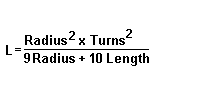# Coil Inductance Calculator

To calculate the inductance of a single-layer, air-core coil:
1. Select the measurement units (inches or centimeters).
2. Enter the number of turns (windings).
3. Enter the coil diameter (form diameter + wire diameter - see diagram).
4. Enter the coil length (distance from first to last winding - see diagram).
5. Click Calculate.

(The following inductance formula requires units in inches.)Turns

Diameter

Length

Depth

Result:

Reference: The ARRL Handbook for Radio Communications 2017